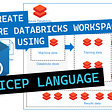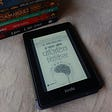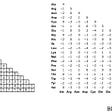# Solution:

• If the counter > 1, we can return an array of the same length as the original array and fill it with zeros.
example: [1,0,2,5,0]
if we cover the 1 = 0*2*5*0 = 0
if we cover the 0 = 1*2*5*0 = 0
etc..
as you can see since we only ever cover one index then there will always be another zero which will always bring the total to 0.
• if the counter =1, then we remember the index of that one zero and and return a new array of the same length filled with zero’s except on the index of where the original zero was located because in that case it means the the total does not zero out since we covered the zero and multiplied all the other numbers.
example: [1,0,2,5]
if we cover the 1 = 0*2*5 = 0
if we cover the 0 = 1*2*5 = 10
if we cover the 2 = 1*0*5 = 0
if we cover the 5 = 1*0*2 = 0
Therefore the array will be filled with zero EXCEPT on the index in which the zero was.
`function arrayOfProducts(array) {  let newArray = []  let num = 1  let counter = 0   let rememberNum 	     if(array.length === 0) {		return array	}	         for(let i = 0; i < array.length; i++) {		if(array[i] === 0) {			counter += 1			rememberNum = i			if(counter > 1) {					                          return new Array(array.length).fill(0);			} 		}          num *= array[i] == 0 ? 1 : array[i]	}	    for(let i = 0; i < array.length; i++) {		if (i == rememberNum){			newArray = new Array(array.length).fill(0);			newArray[i] = num			return newArray		} 		let n = num/array[i]		newArray.push(n)	}	   return newArray}`

--

--

--

## More from Nerly Ton

Love podcasts or audiobooks? Learn on the go with our new app.

## TornadoFX: Adding Ids Dynamically to Control Nodes for TestFX at Runtime## INDUSTRY 5.0: HACKERS RISK## Singleton Design Pattern Explained — A peep into OOP## Create an Azure Databricks workspace using Bicep Language## The first Hackathon I participated.## Kotlin: The Language the Industry Switches to in >5 Years## Build and Deploy a Web-App with React, Flask, NGINX, PostgreSQL, Docker and Google Kubernetes…## GD Studio Reflection/Thoughts on Thesis## The Power Of Positive Thinking By Dr. Norman Vincent Peale |Book Review.## Diverse uses for sequence alignment## SoftBank Operator School (#SBOS) Week 12